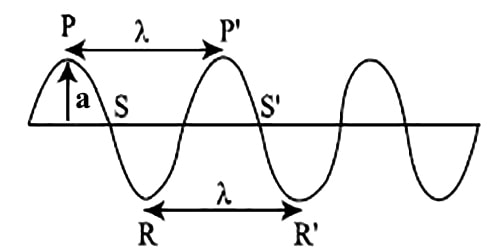Physics

# Explain Wave Related Quantities

Complete vibration: If a wave transmitting particle moves from a certain point and again comes back to the same point then the motion is called complete vibration.

Time period: The time interval in which the wave is repeated that is the times required for one complete vibration of a wave transmitting particle is known as its time period. It is expressed by the letter T and its unit is second (s).

Frequency: The number of complete vibrations in one second of a wave transmitting particle is known as its frequency. Wave is produced from a vibrating object. So the frequency of the vibrating object is equal to the frequency of the wave. The unit of frequency is Hertz (Hz). If a vibrating particle executes one complete vibration in one second then its frequency is called 1 Hz. It is expressed by ‘f’. The relation between frequency and time period is f = 1/T

Amplitude: To produce a wave the particles need to vibrate about their equilibrium position. The maximum displacement of a wave transmitting particle from the position of equilibrium is called amplitude. In the figure ‘a’ is amplitude.Fig: Wave Related Quantities

Phase: The overall condition of motion of a wave transmitting particle at any moment is known as a phase. The overall condition means the displacement, velocity and acceleration etc of the particle at a certain time. The wave crest or the wave trough always remains in the same phase in case of a transverse wave.

In the figure, the particles of points P and P’ or R and R’ are in the same phase.

Wavelength: The distance between the two successive particles of the same phase is called wavelength. Wavelength is defined as the distance through which a wave travels in a time during which a wave transmitting particle completes one oscillation. Wavelength is expressed by γ. Its unit is a meter (m). In the figure, the distance PP’ or RR or SS’ is wavelength, λ.

Wave velocity: The distance that a wave travels in one second in a particular direction is called wave velocity.